# Six pulse bridge converter

## Six pulse bridge converter:

The conduction time of a thyristor in a six pulse connection can be increased when the converter is obtained by a series or parallel connection of two three-pulse midpoint converters. A Six pulse bridge converter is obtained by con­necting two three pulse converters in series on the dc side and in parallel on the ac side. The converter configuration is shown in Fig. 3.34. The three-pulse converter feeding current to the load is called the positive group and the other providing the return path for the current is called the negative group. The star point is no longer necessary, and can be eliminated. There are some obvious advantages when compared to a three-pulse converter: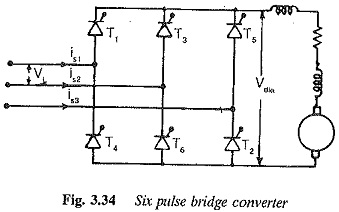1.Because of series connection of the converters on the dc side, the mean output voltage is twice that of a 3 pulse converter for the same supply Consequently the power capability doubles.

2.As no star point is required for the return path, a transformer can be

3.The number of pulses is increased to six and the amplitude of the ac ripple is decreased.

4.The dc component in the secondary of the transformer is completely eliminated. This aspect decreases the design rating of the transformer, if used.

Bridge connections are called two way circuits, since the transformer wind­ings carry current in both directions. This is the reason for eliminating the dc magnetisation of core. On the other hand, midpoint connections have no such facility and thus are one way connections. The bridge connection has thyris­tors conducting for 120° which increases the utilisation of both the thyristors and the transformer, as compared to a Six pulse bridge converter.

The current and voltage waveforms of three-pulse converters connected to form the bridge converter are shown in Fig. 3.35 for different values of the firing angle a. The output voltage of the bridge converter is the algebraic sum of the voltages of the component converters. The positive group has common cathode connection, and will have a thyristor with maximum positive anode voltage conducting. The negative group has a common anode connection and will have a thyristor with maximum negative cathode voltage, conducting. Thus the output voltage at the dc terminals has segments of three phase voltages. Due to the phasedifference between the positive and negative group voltages, the output voltage has a pulse frequency of 6f. The commutations occur alternately in the positive and negative groups. At all times, two thyristors, one in the positive group and the other in the negative group are in conduction. From Fig. 3.35 it is clear that the firing angle can be increased from 0 to 180°. Firing angle α = 0 is the natural firing instant, as has already been defined in the case of a three pulse converter. As the firing angle increases to 90°, the average dc voltage falls to zero. Power flow takes place from ac to dc and the converter is in the rectifying mode. When the angle increases further to 180°, the mean voltage increases from 0 to its maximum value with reversed polarity. If there is a dc source on the load side, power flow takes place from dc to ac and the converter is in the inversion mode. As has already been explained, there is an inverter limit, which is the maximum firing angle (< 180°), beyond which commutation failure occurs in the converter.

The mean voltage at the dc terminals can be shown to be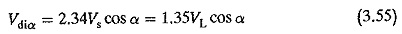where VL is the line to line voltage. The control characteristic is shown in Fig. 3.36.

The peak forward or reverse voltage of a thyristor in a Six pulse bridge converter is the peak value of the line voltage, √2VL. The average current carried by the thyristor is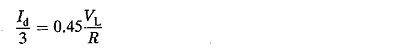The RMS current carried by the thyristor is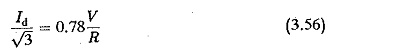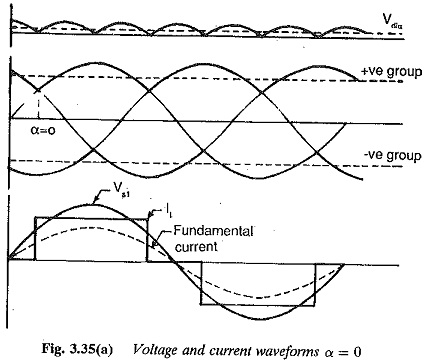The effective value of the secondary current is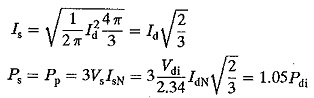It is clear that a bridge circuit is superior compared to a three-pulse or six-pulse connection, in the design rating of the converter transformer.

Considering the effects of overlap, resistance and device drop, the terminal voltage of the converter can be determined in the same way as for a Six pulse bridge converter. It is given by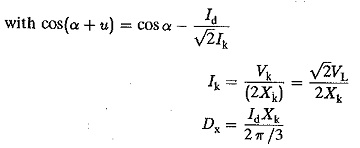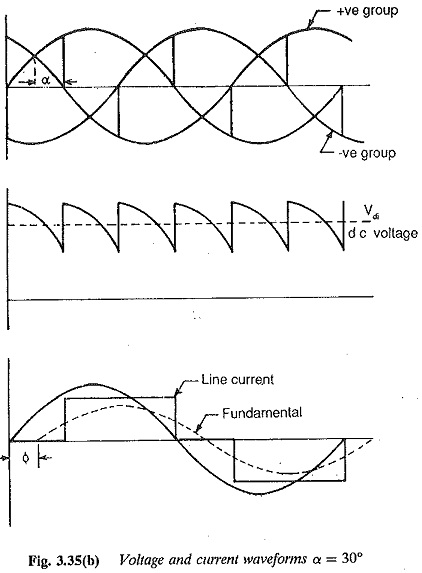The ripple factor of the ac voltage, the smoothing inductance, harmonics in the line current, the power factor and the effects of overlap on these quan­tities can be determined in the same way as for a Six pulse bridge converter. The performance of the converter is shown graphically in Fig. 3.37.Six channels of firing pulses are required, each separated by 60°. During continuous conduction a single pulse is sufficient, whereas in discontinuous conduction each thyristor must be gated for 60°, because an initial starting current is required.